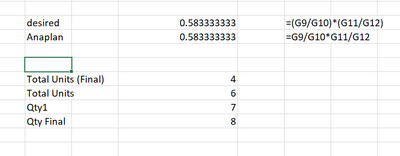# Anaplan Calculation Order of OperationsI am trying to write a simple formula and Anaplan keeps removing the parentheses which causing an issue with the order of operations needed.

What am I missing here? Do I have to break out both parts into separate line items or is there a syntax issue?

Desired: ('Total Units (Final)'  /  Total Units) * ('Qty1' / Qty Final)

Result: 'Total Units (Final)'  /  Total Units * 'Qty1' / Qty Final

Tagged:

•Hi @mooreta ,

Anaplan is probably doing this because results are same in both the case.

Are you seeing any difference in results??

I tried both approaches in spreadsheet and the results seem to be same.Thanks,

Yash

•Hi @mooreta ,

As per my understanding, the simple mathematical order of operations("Operations" mean things like add, subtract, multiply, divide, squaring, etc.), is

 P Parentheses first E Exponents (ie Powers and Square Roots, etc.) MD Multiplication and Division (left-to-right) AS Addition and Subtraction (left-to-right)
• Divide and Multiply rank equally (and go left to right).
• Add and Subtract rank equally (and go left to right)

Thus in your case for the Multiplication and Division the ranking is equal. That can be a reason the Parenthesis are being removed. If you try with some other operations Like Multiplication and Addition then the Parenthesis will not be removed.

Hope this helps.

Thanks ,

Jitendra

•Thank you both. Seems I can't remember 5th grade math well.

I saw the parentheses removed and thought that was the issue. My calculation was incorrect in the first place.

Thanks again!

•Indeed Anaplan removes the un-necessary parenthesis when are used logical or mathematical operators that have the same priority.

I was surprised as well in the first place when I noticed this.

I think this has to do with the optimization of the Anaplan calculation engine in order to parallelize as much as possible.

When parenthesizes are present, the calculation engine is obliged to follow a particular order of the calculation... without them, Anaplan can calculate in parallel (so quicker) some parts of the formula and returning, in the end, the same result.